# Demand surface

## Definition

A demand surface, also known as a joint demand surface, is a geometrical construct that is the analogue, for two goods, of the demand curve for one good. It is ideal for describing situations where the demand for two goods interacts (this could be because they are complementary goods or substitute goods, but it also allows for more complicated relationships).

Explicitly, suppose$A$and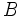$B$ are two goods. The demand surface of$A$and$B$ is a pictorial representation of a function that takes as input the prices of both$A$and$B$, and outputs the quantity demanded for both$A$and$B$. The demand surface graphs this function in four-dimensional space.

If we denote the prices by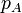$p_A$ and$p_B$ and the quantities by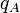$q_A$ and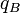$q_B$, the demand surface is the graph of the function: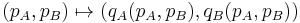$(p_A, p_B) \mapsto (q_A(p_A, p_B), q_B(p_A, p_B))$

The demand surface is two-dimensional and is embedded in four-dimensional space.

## Projections and slices of interest

Slice (intersect with a line, plane, or hyperplane parallel to a coordinate subspace) Projection (project onto a coordinate subspace) Result Dimension of result Dimension of space it is in
No slicing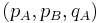$(p_A, p_B, q_A)$ Graph of the function$q_A$ alone, in both of both$p_A$ and$p_B$ 1 3
No slicing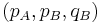$(p_A, p_B, q_B)$ Graph of the function$q_B$ alone, in both of both$p_A$ and$p_B$ 1 3
Fix a value of$p_A$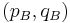$(p_B, q_B)$ Demand curve for$B$ (with specific choice of$p_A$ part of the backdrop) 1 2
Fix a value of$p_A$$(p_B, q_A)$ Cross-demand curve, showing how the quantity demanded for$A$ varies with the price of$B$ (with specific choice of$p_A$ part of the backdrop) 1 2
Fix a value of$p_B$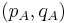$(p_A, q_A)$ Demand curve for$A$ (with specific choice of$p_B$ part of the backdrop) 1 2
Fix a value of$p_B$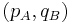$(p_A, q_B)$ Cross-demand curve, showing how the quantity demanded for$B$ varies with the price of$A$ (with specific choice of$p_B$ part of the backdrop) 1 2

## Elasticities

Name of elasticity Formula Sign Explanation
(own-)price-elasticity of demand for$A$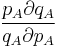$\frac{p_A \partial q_A}{q_A \partial p_A}$ Negative Law of demand for$A$
(own-)price-elasticity of demand for$B$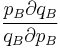$\frac{p_B \partial q_B}{q_B \partial p_B}$ Negative Law of demand for$B$
cross-price elasticity of demand for$B$ with respect to$A$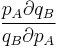$\frac{p_A \partial q_B}{q_B \partial p_A}$ Ambiguous If the goods are complementary goods, it is negative; if they are substitute goods, it is positive
cross-price elasticity of demand for$A$ with respect to$B$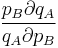$\frac{p_B \partial q_A}{q_A \partial p_B}$ Ambiguous If the goods are complementary goods, it is negative; if they are substitute goods, it is positive

## Cases for the goods

There are three broad cases of interest for the relationship between the goods:

• Complementary goods: This means that an increase in the price of$A$ causes the demand curve for$B$ to move inward (i.e., contract). Similarly, an increase in the price of$B$ causes the demand curve for$A$ to move inward (i.e., contract). Another way of framing it is that the cross-price elasticities of demand (for$A$ with respect to$B$, and for$B$ with respect to$A$) are both negative.
• Substitute goods: This means that an increase in the price of$A$ causes the demand curve for$B$ to move outward (i.e., expand). Similarly, an increase in the price of$B$ causes the demand curve for$A$ to move outward (i.e., expand). Another way of framing it is that the cross-price elasticities of demand (for$A$ with respect to$B$, and for$B$ with respect to$A$) are both positive.
• Independent goods: This means that changes in the price of one good have no effect on the demand curve for the other good. Another way of framing this is that the cross-price elasticities of demand are both zero. In the case of independent goods, the graph of the surface is the Cartesian product of the demand curves for$A$ and$B$.

## Law of joint demand for two goods

Further information: Law of joint demand for two goods

The law of joint demand for two goods says that if we have: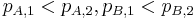$p_{A,1} < p_{A,2}, p_{B,1} < p_{B,2}$

then at least one of these must hold: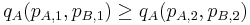$q_A(p_{A,1}, p_{B,1}) \ge q_A(p_{A,2}, p_{B,2})$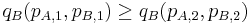$q_B(p_{A,1}, p_{B,1}) \ge q_B(p_{A,2}, p_{B,2})$

In other words, it cannot happen that the quantity demanded increases for both goods when both their prices increase.

When the goods are complementary goods, then in fact, both the above inequalities hold (i.e., both quantities demanded decrease or stay the same). However, when the goods are substitute goods, it is possible for the quantity demanded to increase for one of them when both prices increase. This is because the increase in price of one good pushes some of the demand for it to the other good.

The law of joint demand places a constraint on the nature of the demand surface.